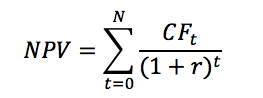Calculating Net Present Value-npv Using Python And Numpy

Overview:

• Net Present Value is a tool for selecting among multiple investment avenues.
• The rule of Net Present Value is to select an investment with a maximum positive NPV and reject the investments that warrant negative NPV or lesser NPVs.
• The formula for NPV isCFt - Net cash flow at time t

N - Total number of Periods

r - Discount rate or rate of return

• Given a series of cash flows the numpy.npv() method returns the Net Present Value of the cash flows.

Example 1: Calculating the NPV for an investment

 import numpy as np   cashflows       = [-500, 200, 147, 128, 130, 235]; # t0, t1, t2, t3, t4, t5 discountRate    = 0.9; # Nine percent per annum npv             = np.npv(discountRate, cashflows);     print("Net present value of the investment:%3.2f"%npv);

Output:

 Net present value of the investment:-315.89